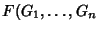## Recursive Function

A recursive function is a function generated by (1) Addition, (2) Multiplication, (3) selection of an element from a list, and (4) determination of the truth or falsity of the Inequalityaccording to the technical rules:

1. Ifand the sequence of functions, ...,are recursive, then so is).

2. Ifis a recursive function such that there is anfor eachwith, then the smallestcan be obtained recursively.
A Turing Machine is capable of computing recursive functions.